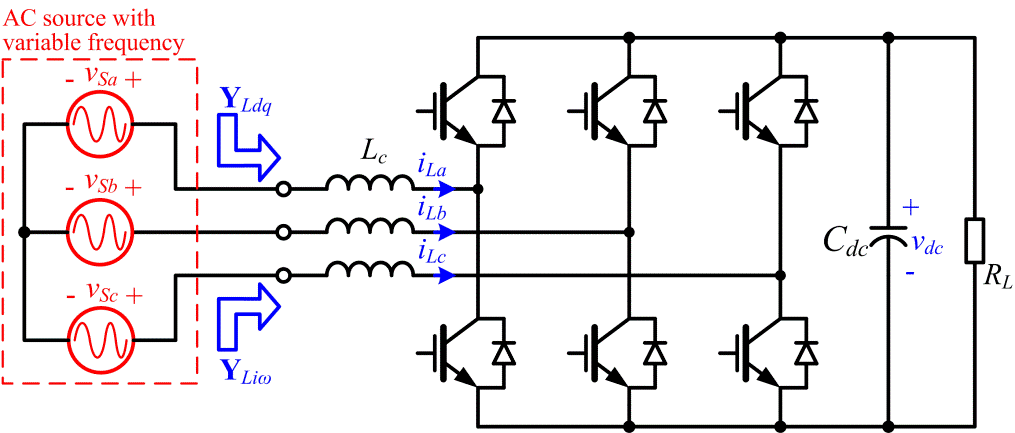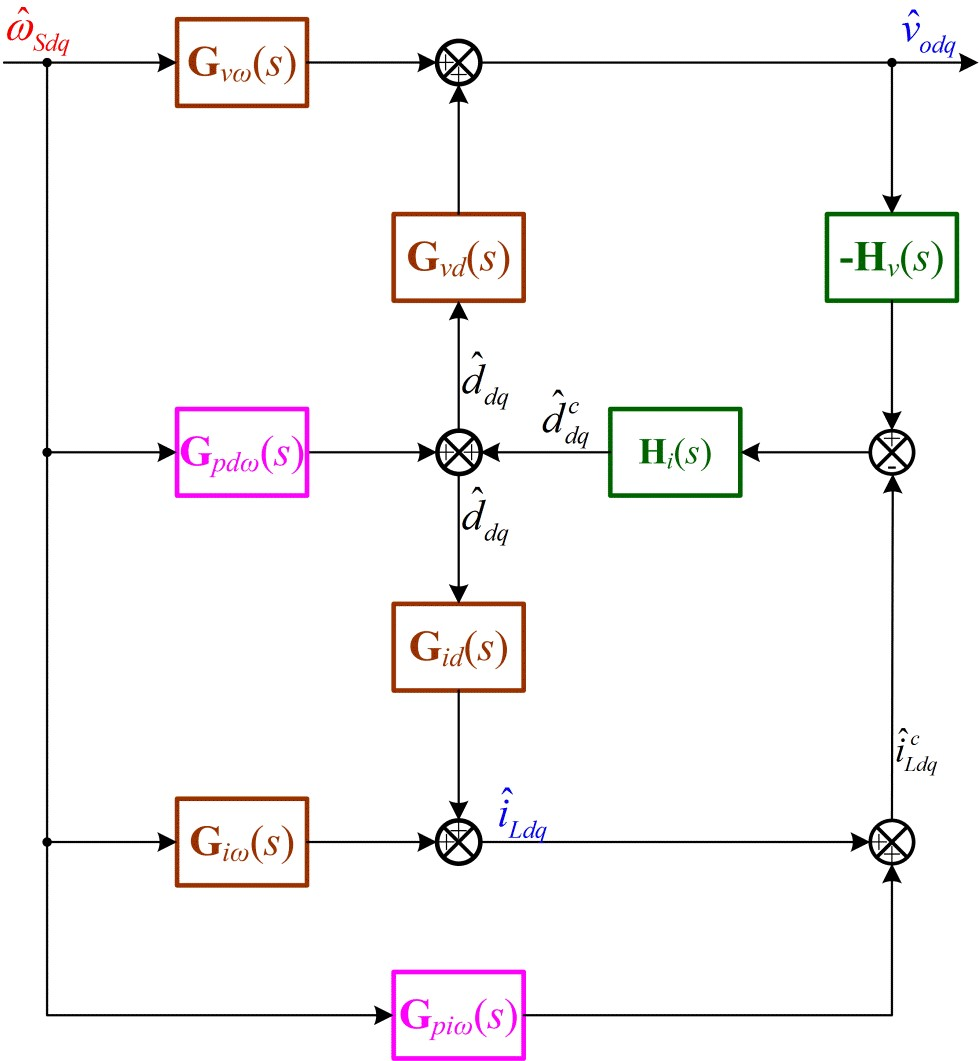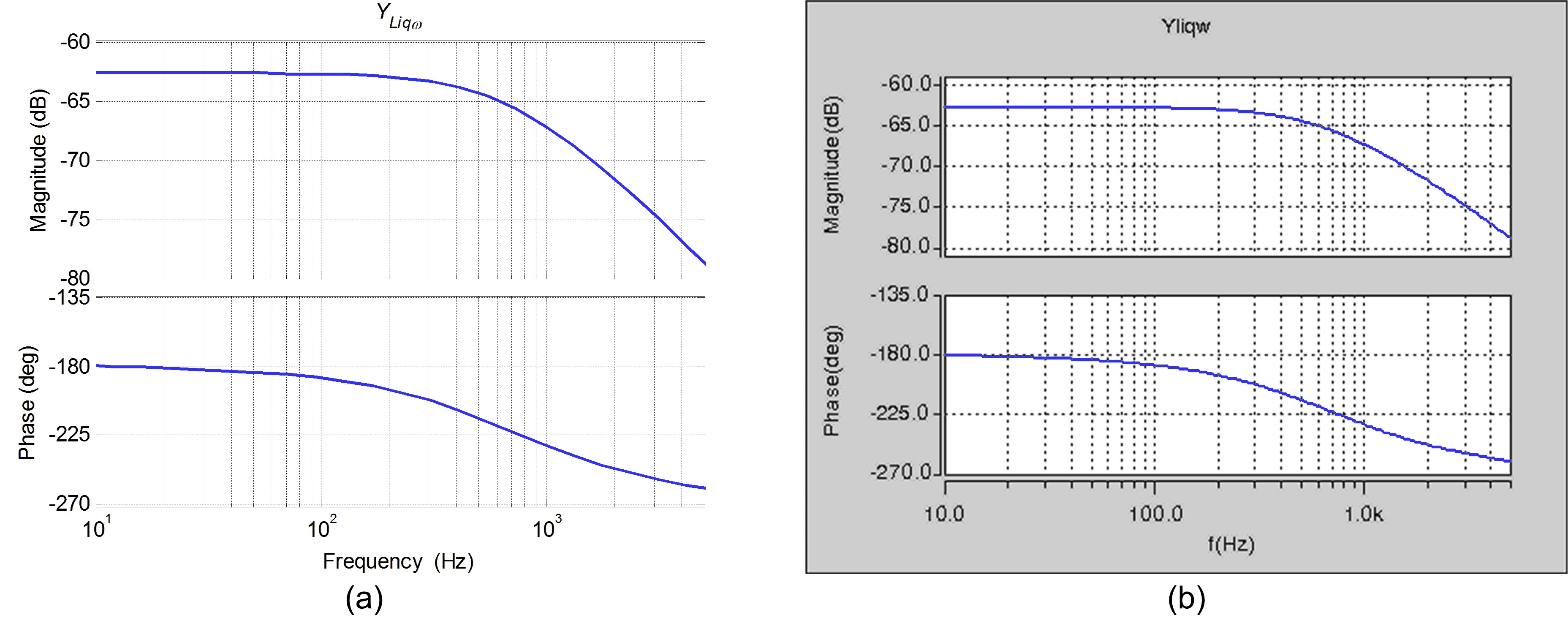LIBRARY

# Wide-bandwidth Identification of Small-Signal dq Impedances of ac Power Sys-tems via Single-Phase Series Voltage Injection

Year: 2016Fig. 1 Power stage of the three-phase boost rectifier studied, where the fundamental frequency of the input voltage is variable.
AC power electronics system are prone to instability due to the interaction of coupling between the power converters, and the terminal characteristics of the individual power converter, which are based on criteria that are very attractive for analyzing system stability. These are represented by a source output impedance and the load input admittance in a synchronous reference frame (SRF) for the conventional three-phase AC system.

In several emerging applications of AC power electronics system, such as micro-grids, the droop control is very popular for power sharing among the parallel power sources, where the droop between active power and fundamental frequency, and the droop between the reactive power and the voltage magnitude are usually implemented. This means that the fundamental frequency of such a system will be varied with the load power, and the coupling between the source and the load not only includes the voltage and the current in SRF, but also covers the fundamental frequency.

As an AFE of the load in the AC power electronics system, the three-phase boost rectifier in Fig. 1 is widely employed, and thus its terminal characteristics regarding the variation of fundamental frequency are studied. Its small-signal model considering the dynamic behavior of the fundamental frequency is proposed as shown in Fig. 2. Here, it is revealed that the dynamic of fundamental frequency is introduced by both the fundamental voltage drop of the filter inductor and the PLL. The transfer function of the terminal characteristics from fundamental frequency to input current in SRF is derived, and verified in frequency domain. These bodeplots are partially shown in Fig. 3. The proposed model can be applied for the stability analysis of the system with variable fundamental frequency.Fig. 2. Small-signal model of the three-phase boost rectifier in SRF covering the dynamic of fundamental frequency.Fig. 3 Bode plot of transfer function from fundamental frequency to Q axis input current: (a) model, (b) measurement.